### MEMO 2011 pojedinačno problem 1

Kvaliteta:
Avg: 4,0
Težina:
Avg: 6,0
Initially, only the integer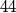$44$ is written on a board. An integer a on the board can be re- placed with four pairwise different integers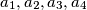$a_1, a_2, a_3, a_4$ such that the arithmetic mean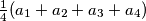$\frac 14 (a_1 + a_2 + a_3 + a_4)$ of the four new integers is equal to the number$a$. In a step we simultaneously replace all the integers on the board in the above way. After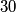$30$ steps we end up with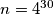$n = 4^{30}$ integers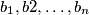$b_1, b2,\ldots, b_n$ on the board. Prove that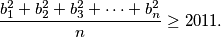Izvor: Srednjoeuropska matematička olimpijada 2011, pojedinačno natjecanje, problem 1